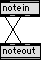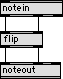# Digital Music Programming II: flip

This lab demonstrates how to switch the MIDI key number and MIDI attack velocity so that the velocity controls pitch and the pitch controls velocity.

A simple method of doing this is demonstrated in the following patch:Try out this patch. Whenever you play a loud note, a high note will occur. Whenever you play a soft note, a low pitch will occur. Also, if you play a high pitch, the resulting note will be loud, and if you play a low note, the resulting pitch will be soft.

There is a bug in the above patch. Listen to a performance with the above patch and determine what the bug is.

The following external object called flip flip fixes the bug.

There are two inputs and two outputs for the flip object:

 Inputs Outputs 1. MIDI Note Number 1. MIDI Note Number (from input 2) 2. MIDI Attack Velocity 2. MIDI Attack Velocity (from input 1)

# Source Code

The first program below shows how to generate a monophonic flip program. One note can be played at a time and not develope any bugs.

monoflip.c
 ``` #include "ext.h" typedef struct { t_object max_data; long keyout; long velin; void* outputKeyNumber; void* outputVelocity; } MyObject; void* object_data = NULL; void* create_object (void); void InputKeyNumber (MyObject* mo, long value); void InputVelocity (MyObject* mo, long value); void main(void) { setup((t_messlist**)&object_data, (method)create_object, NULL, sizeof(MyObject), NULL, A_NOTHING); addint ((method)InputKeyNumber); addinx ((method)InputVelocity, 1); } void* create_object(void) { int i; MyObject* mo = (MyObject*)newobject(object_data); mo->velin = 0; for (i=0; i<128; i++) mo->keymap[i] = 0; mo->outputVelocity = intout(mo); mo->outputKeyNumber = intout(mo); intin(mo, 1); return mo; } void InputKeyNumber(MyObject* mo, long value) { if (mo->velin == 0) { outlet_int(mo->outputVelocity, mo->velin); outlet_int(mo->outputKeyNumber, mo->keyout); } else { outlet_int(mo->outputVelocity, value); outlet_int(mo->outputKeyNumber, mo->velin); mo->keyout = mo->velin; } } void InputVelocity(MyObject* mo, long value) { mo->velin = value; } ```

Here is a fully polyphonic flip object which does not exhibit the bug from monoflip.c or the plain notein-noteout patch. Compare performance using this object with the one above.

polyflip.c
 ``` #include "ext.h" typedef struct { t_object max_data; long keymap; long velin; void* outputKeyNumber; void* outputVelocity; } MyObject; void* object_data = NULL; void* create_object (void); void InputKeyNumber (MyObject* mo, long value); void InputVelocity (MyObject* mo, long value); long midilimit (long value); void main(void) { setup((t_messlist**)&object_data, (method)create_object, NULL, sizeof(MyObject), NULL, A_NOTHING); addint ((method)InputKeyNumber); addinx ((method)InputVelocity, 1); } void* create_object(void) { int i; MyObject* mo = (MyObject*)newobject(object_data); mo->velin = 0; for (i=0; i<128; i++) mo->keymap[i] = 0; mo->outputVelocity = intout(mo); mo->outputKeyNumber = intout(mo); intin(mo, 1); return mo; } void InputKeyNumber(MyObject* mo, long value) { value = midilimit(value); if (mo->velin == 0) { outlet_int(mo->outputVelocity, mo->velin); outlet_int(mo->outputKeyNumber, mo->keymap[value]); } else { outlet_int(mo->outputVelocity, value); outlet_int(mo->outputKeyNumber, mo->velin); mo->keymap[value] = midilimit(mo->velin); } } void InputVelocity(MyObject* mo, long value) { mo->velin = value; } long midilimit(long value) { if (value < 0) return 0; if (value > 127) return 127; return value; } ```

Why is the midilimit function in polyflip.c necessary?

# Exercises

1. Compile the two flip objects and test them with the following patch:using a sustained instrument such as organ.

2. Write a Max patch which has the same behavior as the flip object. Note that the following patch does not have the same behavior:3. Write a program which mirrors the notes rather than switches the key numbers and velocities. Add an input to the object for the mirror position.

```

```